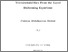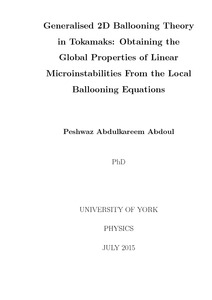# Generalised 2D ballooning theory in tokamaks: obtaining the global properties of linear microinstabilities from the local ballooning equations

Abdoul, Peshwaz (2015) Generalised 2D ballooning theory in tokamaks: obtaining the global properties of linear microinstabilities from the local ballooning equations. PhD thesis, University of York.Preview
Text (Generalised 2D ballooning theory in tokamaks)
thesis.pdf - Examined Thesis (PDF)
This thesis involves the development of a technique to improve our understanding of the impact of global effects on linear microinstabilities. The technique combines local ballooning solutions with higher order theory to reconstruct the global eigenmode structure and associated global eigenvalue. In this work, the theory of 2D ballooning eigenmodes has been extended beyond the two limits of highly unstable isolated modes, which often sit on the outboard mid-plane, and less unstable general modes that peak on the top or bottom of the tokamak. Our generalised solutions can account for global modes with arbitrary poloidal positions which, in turn, enables us to explore experimentally relevant regimes with arbitrary equilibria. Furthermore, using a generally applicable gyrokinetic plasma model, the global mode structures of the ion temperature gradient (ITG) driven microinstability have been reconstructed directly from the local gyrokinetic code, GS2. Using CYCLONE base case parameters with the circular Miller equilibrium model we benchmark our calculation against direct global gyrokinetic simulations. Introducing a radially varying profile for the mode drive that peaks about a reference rational surface $x_{s}=0$, while holding other profiles constant, leads to a highly unstable isolated mode. The effect of the other equilibrium profiles is found to be stabilising. However, rotational flow shear introduced via a Doppler shift in the real local frequency, i.e $\omega_{0} \rightarrow \omega_{0} + n\qprime\gamma_{E} x_{s}$, where $n$ is the toroidal mode number and $\gamma_{E}$ represents the strength of the flow shear, can cancel the influence of the other profile variations and, for a critical value of flow shear, a highly unstable isolated mode can be captured again. Finally, for an equilibrium that exhibits an isolated mode, we have also investigated the effect of the flux surface shaping through elongation $\bar{\kappa}$ and triangularity $\bar{\delta}$. Our simulations indicate that, depending on the value of $\bar{\kappa}$ and $\bar{\delta}$, the effect of shaping can be both stabilising and destabilising.